EnchantedLearning.com is a user-supported site.
As a bonus, site members have access to a banner-ad-free version of the site, with print-friendly pages.

 More MathActivities EnchantedLearning.comFractions NUMERATORDENOMINATOR
 IdentifyingFractions Reducing toSimplest Form,Equivalent Fractions ImproperFractions Fractions toDecimals, Percents ComparingFractions AddingFractions MultiplyingFractions Worksheetsand Booksto Print SubtractingFractions DividingFractions
 Overview of Fractions "…Bliss and envy are the numerator and denominator of the fraction called happiness." ~Yevgeny Zamyatin

A fraction is a part of a whole, like one half (1/2), one third (1/3), two thirds (2/3), one quarter (1/4), etc. For example, half of an apple is a fraction of an apple.

A fraction can be expressed in the form a/b, where the top number, a, is called the numerator; the bottom number, b, is called the denominator. The denominator of a fraction cannot be equal to zero (this is because you cannot divide a number by zero).

The value of a fraction is not changed if both the numerator and denominator are multiplied or divided by the same non-zero number.

 Equivalent Fractions:Equivalent fractions are different fractions that are equal to the same number (like 2/4 and 3/6) and can be simplified and written as the same fraction (1/2 in this example).
 Expressing Fractions in Simplest Form:A fraction is in its simplest form (this is also called being expressed in lowest terms) if the Greatest Common Factor (GCF), also called the Greatest Common Denominator (GCD), of the numerator and denominator is 1. To reduce a fraction to lowest terms (also called its simplest form), divide both the numerator and denominator by the GCD. For example, 2/3 is in lowest form, but 4/6 is not in lowest form (the GCD of 4 and 6 is 2) and 4/6 can be expressed as 2/3.
 Improper Fractions: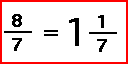An improper fraction is one whose numerator is larger than the denominator (like 5/4). Improper fractions can be written as a mixed number, a number plus a fraction (for example, 5/4 = 1 1/4).
 Fractions Written as Decimals:Every fraction can be expressed as decimal (a number with a decimal point). One way to convert a fraction to a decimal is to divide the numerator by the denominator. For example, 1/2 is equal to 1 divided by 2, which is equal to 0.5.
 Fractions to Percents: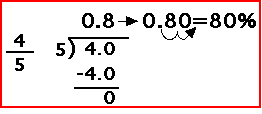A fraction can be expressed as a percentage by converting the fraction to a decimal number, then multiplying by 100 to obtain a percentage (since percent means per 100). For example, 1/2 = 0.5 = 50%.
 Adding Fractions:To add two or more fractions, first make all the fractions have the same denominator. Once the denominators are the same, just add the numerators. Then simplify the answer (put the fraction in lowest terms).
 Multiplying Fractions: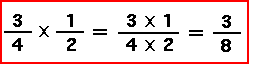To multiply two or more fractions, multiply the numerators and multiply the denominators. Then simplify the answer (put the fraction in lowest terms).
 Comparing Fractions:To compare two fractions (that is, to find out which one is bigger or smaller), first write the fractions so that they have the same denominator. Then, compare the numerators; the fraction with the largest positive numerator is the larger fraction.

Fraction Worksheets:
Introduction to Fractions - Worksheets and Games to Print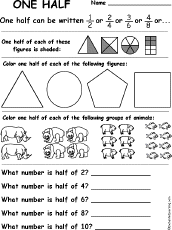One Half: A Fraction WorksheetLearn about the fraction one half and do simple problems on 1/2. Or go to the answers.One Third: A Fraction WorksheetLearn about the fraction one third and do simple problems on 1/3. Or go to the answers.One Quarter: A Fraction WorksheetLearn about the fraction one quarter and do simple problems on 1/4. Or go to the answers.One Fifth: A Fraction WorksheetLearn about the fraction one fifth and do simple problems on 1/5. Or go to the answers.Printable Set of Fraction DominoesPrint a set of fractions dominoes; each tile has fractions on it (1/2, 1/3, 1/4, 1/5, 1/6, 2/3, and 3/4) in words, in reduced form, as equivalent fractions, and in pictures. In this game the student matches fractions in different forms.Color Fractions of Groups of Animals WorksheetColor halves, thirds, quarters and fifths of the groups of fish, turtles, frogs, and lizards.Color Fractions of Groups of Shapes WorksheetColor halves, thirds, quarters and fifths of groups of squares, triangles, circles, and ovals.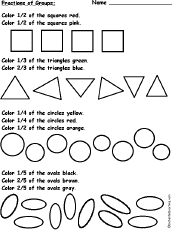Color Fractions of Groups of Shapes Worksheet #2Color halves, thirds, quarters and fifths of groups of squares, triangles, circles, and ovals.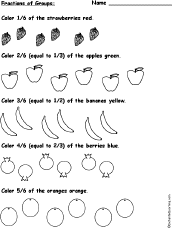Color Fractions of Groups of Fruit - Worksheet on SixthsColor 1/6, 2/6=1/3, 3/6=1/2, 4/6=2/3, and 5/6 of groups of strawberries, apples, bananas, berries, and oranges.Color Fractions of Groups of Fruit - Worksheet on EighthsColor 1/8, 2/8=1/4, 3/8, 4/8=1/2, 5/8, 6/8=3/4, 5/8 of groups of limes, apples, pears, berries, oranges, and cherries.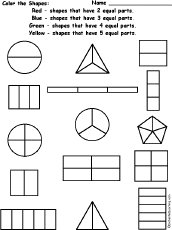Color Fractions WorksheetColor the Divided Shapes: Red - shapes that have 2 equal parts. Blue - shapes that have 3 equal parts. Green - shapes that have 4 equal parts. Yellow - shapes that have 5 equal parts.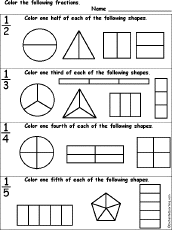Color Fractions WorksheetColor 1/2, 1/3, 1/4, or 1/5 of the pictures.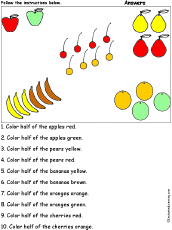Food Fractions #1: Follow the InstructionsDraw and color fractions (one half) of groups of fruit according to simple directions, such as, "Color one half of the apples red." Or go to the answers.Food Fractions #2: Follow the InstructionsDraw and color fractions according to simple directions, such as, "Color one third of the candies purple." Or go to the answers.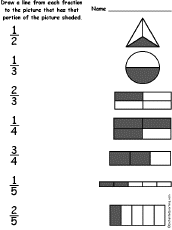Match Fractions WorksheetDraw a line from each fraction to the picture that has that portion of the picture shaded. Or go to the answers.Match Fractions Worksheet #2Draw a line from each fraction to the picture that has that portion of the picture shaded. Or go to the answers.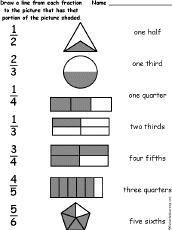Match Fractions Worksheet #3Draw a line from each fraction (written in fraction form and in English) to the picture that has that portion of the picture shaded. Or go to the answers.Fractions of Robots PrintoutFind the fractions of the robots that are made of different shapes. Or go to the answers.Fractions of Groundhogs PrintoutFind the fractions of the groundhogs with and with a shadow, in and out of a burrow, looking to the right or left, facing their shadow, etc. Or go to the answers.Fractions of Balloons PrintoutFind the fractions of the balloons that have different patterns. Or go to the answers.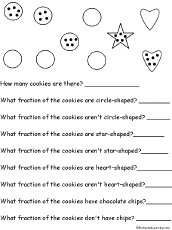Fractions of Cookies PrintoutFind the fractions of the cookies that have different shapes and chocolate chips or not. Or go to the answers.Fractions of Ice Cream Cones PrintoutFind the fractions of the ice cream cones that have different numbers of scoops, all vanilla ice cream, or a cherry on top. Or go to the answers.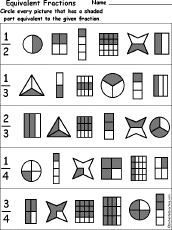Equivalent Fractions WorksheetCircle every picture that has a shaded part equivalent to the given fraction, for 1/2, 1/3, 2/3, 1/4, and 3/4. Or go to the answers.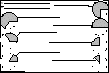FractionsLabel Me! PrintoutLabel the fractions.Answers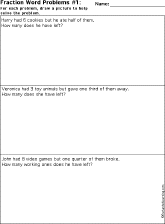Word Problems: FractionsDraw a picture or use an equation to help you solve each fraction problem. Worksheet #1. Answers: 3, 2, 6 Worksheet #2. Answers: 5, 3, 1 Worksheet #3. Answers: 4, 4, 1 Worksheet #4. Answers: 9, 6, 1 Worksheet #5. Answers: 6, 8, 3 Worksheet #6. Answers: 8, 2, 2 Worksheet #7. Answers: 3, 9, 3 Worksheet #8. Answers: 7, 2, 10 Identifying Fractions Word ProblemsSolve word problems that involve identifying fractions. Draw pictures to help solve these word problems. Shading Fractions of Figures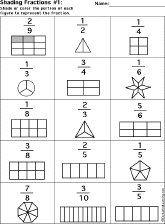Shade or color the portion of each figure to represent the fraction. Shade and Reduce Fractions of Figures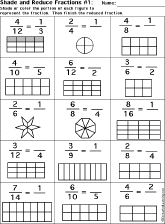Shade or color the portion of each figure to represent the fraction. Then finish writing the fraction in reduced terms.FractionsPrintable Number BookA short, printable book for early readers, about fractions, with pages for the student to cut out, items to color, and short phrases to copy.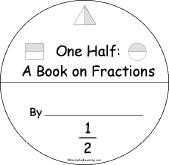One HalfPrintable Fraction BookA short, printable book about the fraction one half for early reader, with pages for the student to cut out, items to color, fraction activities, and short phrases to copy.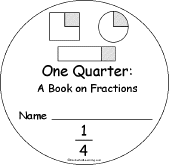One QuarterPrintable Fraction BookA short, printable book about the fraction one quarter for early reader, with pages for the student to cut out, items to color, fraction activities, and short phrases to copy.Pumpkin Patch: FractionsPrintable BookA short, printable book about fractions of fall items, including pumpkins, scarecrows, pies, haystacks, apples, leaves, acorns, ears of corn, and spiders.Spring FractionsPrintable BookA short, printable book about fractions of spring items, including butterflies, birds, eggs, caterpillars, flowers, tadpoles, rogs, ducks, and rabbits.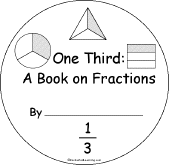One ThirdPrintable Fraction BookA short, printable book about the fraction one third for early reader, with pages for the student to cut out, items to color, fraction activities, and short phrases to copy.

Fraction Worksheets:
 Writing Fractions and Reducing to Lowest TermsFor each geometric figure, write the fraction of each figure that is dark. Then express the fraction in lowest terms. Reduce Fractions to Lowest Terms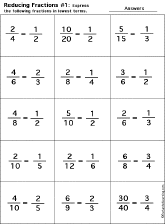Each worksheet has 15 fractions to reduce to lowest terms (also called expressing in lowest terms). To reduce a fraction to lowest terms, divide both the numerator and denominator by the Greatest Common Divisor (GCD) (also called the Greatest Common Factor (GCF)). Express Improper Fractions as Mixed NumbersEach worksheet has improper fractions to express as mixed numbers. Express Mixed Numbers as Improper Fractions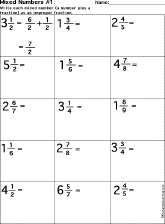Each worksheet has mixed numbers to express as improper fractions. Express Fractions as Decimals and as Percents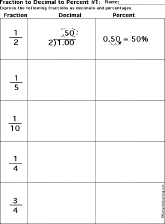Each worksheet has fractions to express as decimals and percentages. Express Decimals as Fractions and as PercentsEach worksheet has decimals to express as fractions and percentages. Express Percents as Fractions and Decimals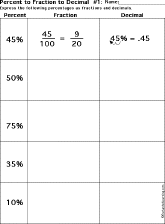Each worksheet has percentages to convert to decimals and fractions. Express Fractions as Percents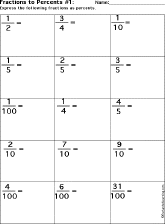Each worksheet has 15 fractions to express as percents. Adding Fractions With the Same Denominators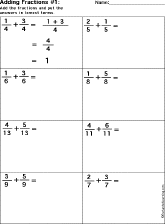Add the fractions (they have the same denominators). Drawing and Adding Fractions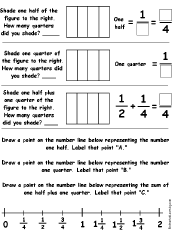Draw and add two fractions and plot them on a number line. Adding Fractions With Different DenominatorsAdd the fractions (they have different denominators). Subtracting Fractions With the Same Denominators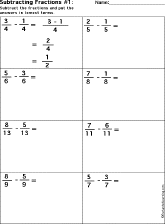Subtract the fractions (they have the same denominators). Subtracting Fractions With Different DenominatorsSubtract the fractions (they have different denominators). Fractions of an HourFor each of the questions, list the time and draw the hands on the clock to show the time. Subtracting Fractions Word ProblemsSolve word problems that involve subtracting fractions. Draw pictures to help solve these word problems. Multiplying Fractions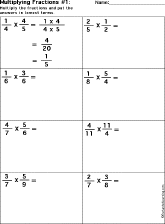Multiply the fractions. Dividing FractionsDivide the fractions.LCM, GCDFactor two numbers into primes, then use a Venn diagram to compute their Least Common Multiple (LCM) and Greatest Common Divisor (GCD). Or go to an example of how to use this diagram. Comparing Fractions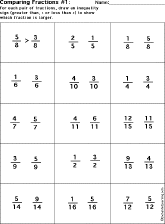Compare the pairs of fractions, determining which is larger.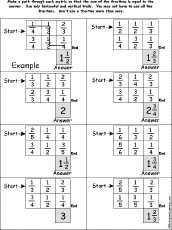Adding Fractions Path-o-Math Puzzles: 2 by 3Make a path through each number matrix so that the sum of the fractions is equal to the given answer. Use only horizontal and vertical trails. You may not have to use all the numbers, and don't use a number more than once. Puzzle #1, or the answers Puzzle #2, or the answers Puzzle #3, or the answers Puzzle #4, or the answers Puzzle #5, or the answers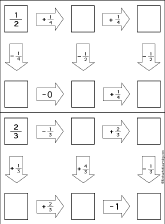Addition and Subtraction of Fractions Follow-the-Arrows Puzzles Do addition and subtraction of fractions along the paths according to the arrows until you get to the end. You should get to the same final answer three different ways. Puzzle #1, or the answers Puzzle #2, or the answers Puzzle #3, or the answers Puzzle #4, or the answers Puzzle #5, or the answersMultiplication of Fractions Follow-the-Arrows Puzzles Do multiplication of fractions along the paths according to the arrows until you get to the end. You should get to the same final answer three different ways. Puzzle #1, or the answers Puzzle #2, or the answers Puzzle #3, or the answers Puzzle #4, or the answers Puzzle #5, or the answers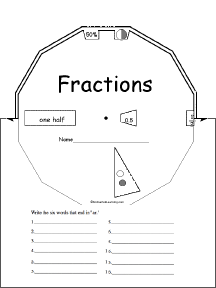Fractions WheelMake a wheel about fractions using this 2-page print-out; it consists of a base page together with a wheel that spins around. When you spin the wheel, fractions appear (including the fraction written out in English, as a numerator and deniminator, in decimal form, as a percent, illustrated as a pie diagram, and illustrated as a portion of a series of circles). The fractions are one-half, one-quarter, one-third, two-thirds, one-sixth, two-fifths, three-quarters, one-fifth, one-eighth, three-fifths, one-tenth, and four-fifths. The student then writes down the fractions on the wheel in order from smallest to biggest.

 +, - EnchantedLearning.comMath x, ÷
 A B C D E F G H I J K L M N O P Q R S T U V W X Y Z
 Number Line Fractions Decimals Measurement Rounding Graphs

Enchanted Learning®
Over 35,000 Web Pages
Sample Pages for Prospective Subscribers, or click below

 Overview of Site What's New Enchanted Learning Home Monthly Activity Calendar Books to Print Site Index K-3 Crafts K-3 Themes Little ExplorersPicture dictionary PreK/K Activities Rebus Rhymes Stories Writing Cloze Activities Essay Topics Newspaper Writing Activities Parts of Speech Fiction The Test of Time Biology Animal Printouts Biology Label Printouts Biomes Birds Butterflies Dinosaurs Food Chain Human Anatomy Mammals Plants Rainforests Sharks Whales Physical Sciences: K-12 Astronomy The Earth Geology Hurricanes Landforms Oceans Tsunami Volcano Languages Dutch French German Italian Japanese (Romaji) Portuguese Spanish Swedish Geography/History Explorers Flags Geography Inventors US History Other Topics Art and Artists Calendars College Finder Crafts Graphic Organizers Label Me! Printouts Math Music Word Wheels

## Enchanted Learning Search

 Search the Enchanted Learning website for: